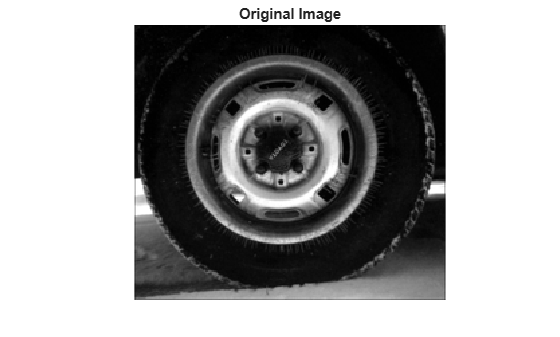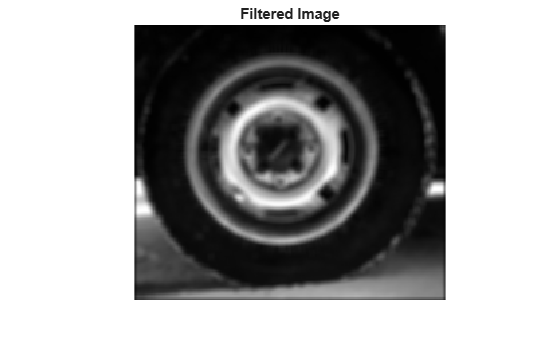# colfilt

Column-wise neighborhood operations

## Syntax

``B = colfilt(A,[m n],block_type,fun)``
``B = colfilt(A,[m n],[mblock nblock],block_type,fun)``
``B = colfilt(A,'indexed',___)``

## Description

example

````B = colfilt(A,[m n],block_type,fun)` processes the image `A` by rearranging each `m`-by-`n` block of `A` into a column of a temporary matrix, and then applying the function `fun` to this matrix. `colfilt` zero-pads `A`, if necessary.```
````B = colfilt(A,[m n],[mblock nblock],block_type,fun)` subdivides `A` into regions of size `mblock`-by-`nblock` blocks to save memory. Note that the result of the operation does not change when using the `[mblock nblock]` argument.For example, if `[mblock nblock]` is `[3 4]` and the size of each block is 16-by-16 pixels, then `colfilt` subdivides the image into regions of size 48-by-64 pixels and processes each region separately.```
````B = colfilt(A,'indexed',___)` processes `A` as an indexed image, padding with `0`s if the class of `A` is `uint8`, `uint16`, or `logical`, and padding with `1`s otherwise.```

## Examples

collapse all

This example shows how to set each output pixel to the mean value of the input pixel's 5-by-5 neighborhood using column-wise neighborhood processing.

Read a grayscale image into the workspace.

`I = imread('tire.tif');`

Perform column-wise filtering. The function `mean` is called on each 5-by-5 pixel neighborhood.

`I2 = uint8(colfilt(I,[5 5],'sliding',@mean));`

Display the original image and the filtered image.

```imshow(I) title('Original Image')``````figure imshow(I2) title('Filtered Image')```## Input Arguments

collapse all

Image, specified as an array of any class supported by `fun`.

Block size, specified as a 2-element vector of positive integers. `m` is the number of rows and `n` is the number of columns in each block.

Block group size, specified as a 2-element vector of positive integers. `mblock` is the number of blocks in the group in the vertical direction, and `nblock` is the number of blocks in the group in the horizontal direction.

Block type, specified as `'sliding'` for sliding neighborhoods or `'distinct'` for distinct blocks.

Data Types: `char` | `string`

Function handle, specified as a handle. The input and output arguments to this function depend on the value of `block_type`. For more information, see Algorithms.

## Output Arguments

collapse all

Filtered image, returned as a numeric matrix.

## Algorithms

The algorithm that `colfilt` uses to process images depends on the value of `block_type`.

Value

Description

`'distinct'`

• First, `colfilt` rearranges each `m`-by-`n` block of `A` into a column in a temporary matrix by using the `im2col` function.

• Next, `colfilt` applies the function `fun` to this temporary matrix. `fun` must return a matrix the same size as the temporary matrix.

• Finally, `colfilt` rearranges the columns of the matrix returned by `fun` into `m`-by-`n` distinct blocks, by using the `col2im` function.

`'sliding'`

• First, `colfilt` rearranges each `m`-by-`n` neighborhood of `A` into a column in a temporary matrix by using the `im2col` function.

• Next, `colfilt` applies the function `fun` to this temporary matrix. `fun` must return a row vector containing a single value for each column in the temporary matrix. (Column compression functions such as `sum` return the appropriate type of output.)

• Finally, `colfilt` reshapes the vector returned by `fun` into a matrix the same size as `A`, by using the `reshape` function.

To save memory, the `colfilt` function might divide `A` into subimages and process one subimage at a time. This implies that `fun` may be called multiple times, and that the first argument to `fun` may have a different number of columns each time.

Introduced before R2006a